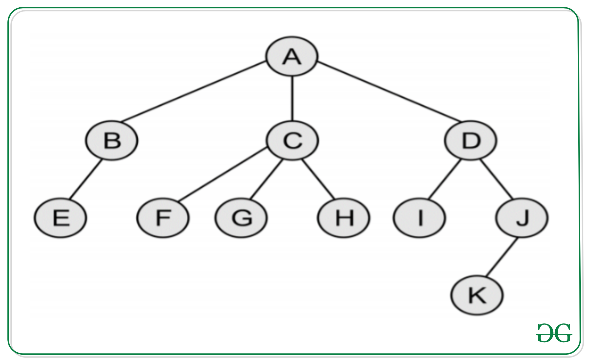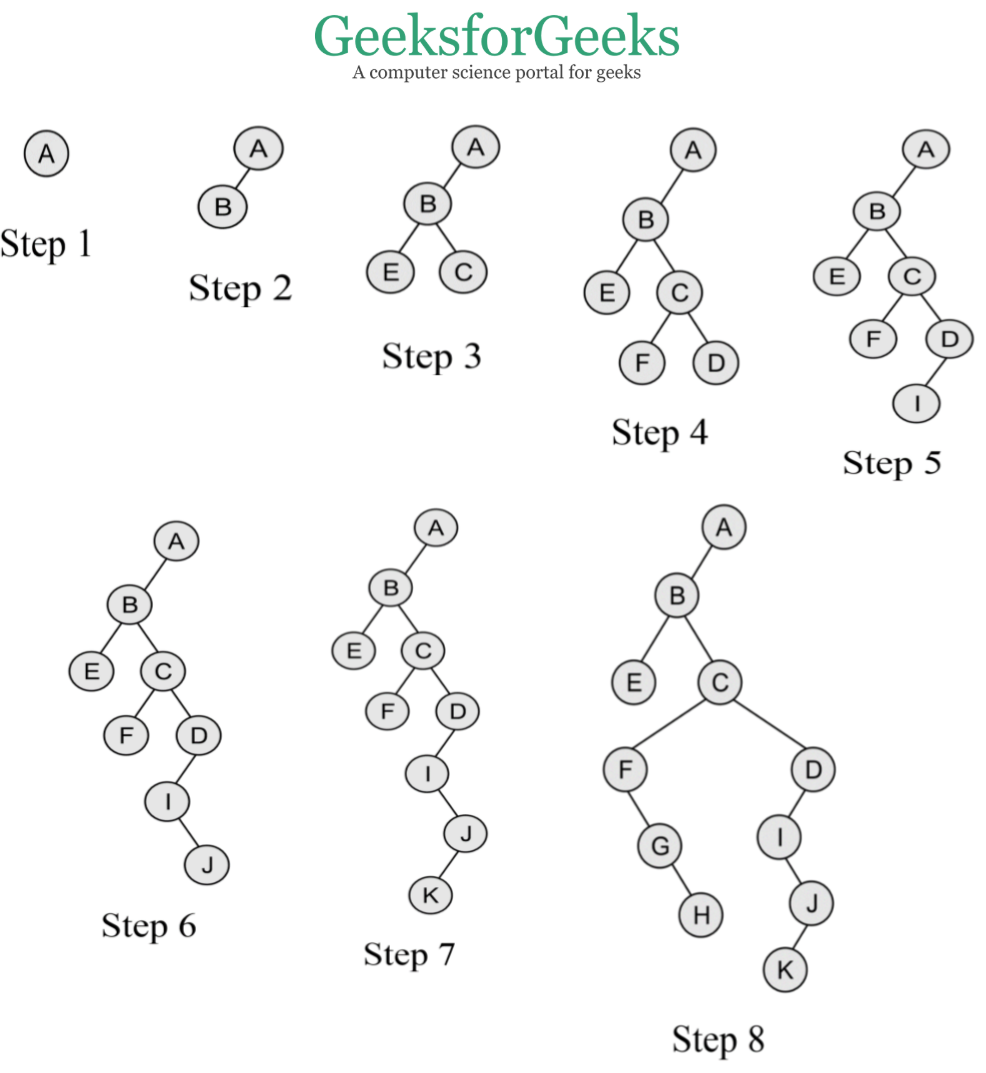GeeksforGeeks App
Open AppBrowser
Continue

# Convert a Generic Tree(N-array Tree) to Binary Tree

Prerequisite: Generic Trees(N-array Trees)

In this article, we will discuss the conversion of the Generic Tree to a Binary Tree. Following are the rules to convert a Generic(N-array Tree) to a Binary Tree:

• The root of the Binary Tree is the Root of the Generic Tree.
• The left child of a node in the Generic Tree is the Left child of that node in the Binary Tree.
• The right sibling of any node in the Generic Tree is the Right child of that node in the Binary Tree.

Examples:
Convert the following Generic Tree to Binary Tree:Below is the Binary Tree of the above Generic Tree:Note: If the parent node has only the right child in the general tree then it becomes the rightmost child node of the last node following the parent node in the binary tree. In the above example, if node B has the right child node L then in binary tree representation L would be the right child of node D.

Below are the steps for the conversion of Generic Tree to Binary Tree:

1. As per the rules mentioned above, the root node of general tree A is the root node of the binary tree.
2. Now the leftmost child node of the root node in the general tree is B and it is the leftmost child node of the binary tree.
3. Now as B has E as its leftmost child node, so it is its leftmost child node in the binary tree whereas it has C as its rightmost sibling node so it is its right child node in the binary tree.
4. Now C has F as its leftmost child node and D as its rightmost sibling node, so they are its left and right child node in the binary tree respectively.
5. Now D has I as its leftmost child node which is its left child node in the binary tree but doesn’t have any rightmost sibling node, so doesn’t have any right child in the binary tree.
6. Now for I, J is its rightmost sibling node and so it is its right child node in the binary tree.
7. Similarly, for J, K is its leftmost child node and thus it is its left child node in the binary tree.
8. Now for C, F is its leftmost child node, which has G as its rightmost sibling node, which has H as its just right sibling node and thus they form their left, right, and right child node respectively.

## C++

 `#include ``#include ` `class` `TreeNode {``public``:``    ``int` `val;``    ``TreeNode* left;``    ``TreeNode* right;``    ``std::vector children;``    ``TreeNode(``int` `val)``    ``{``        ``this``->val = val;``        ``this``->left = ``this``->right = nullptr;``    ``}``};` `TreeNode* convert(TreeNode* root)``{``    ``if` `(!root) {``        ``return` `nullptr;``    ``}` `    ``if` `(root->children.size() == 0) {``        ``return` `root;``    ``}` `    ``if` `(root->children.size() == 1) {``        ``root->left = convert(root->children);``        ``return` `root;``    ``}` `    ``root->left = convert(root->children);``    ``root->right = convert(root->children);` `    ``for` `(``int` `i = 2; i < root->children.size(); i++) {``        ``TreeNode* rightTreeRoot = root->right;``        ``while` `(rightTreeRoot->left != nullptr) {``            ``rightTreeRoot = rightTreeRoot->left;``        ``}``        ``rightTreeRoot->left = convert(root->children[i]);``    ``}` `    ``return` `root;``}` `void` `printTree(TreeNode* root)``{``    ``if` `(!root) {``        ``return``;``    ``}``    ``std::cout << root->val << ``" "``;``    ``printTree(root->left);``    ``printTree(root->right);``}` `int` `main()``{``    ``TreeNode* root = ``new` `TreeNode(1);``    ``root->children.push_back(``new` `TreeNode(2));``    ``root->children.push_back(``new` `TreeNode(3));``    ``root->children.push_back(``new` `TreeNode(4));``    ``root->children.push_back(``new` `TreeNode(5));` `    ``root->children->children.push_back(``new` `TreeNode(6));``    ``root->children->children.push_back(``new` `TreeNode(7));``    ``root->children->children.push_back(``new` `TreeNode(8));``    ``root->children->children.push_back(``new` `TreeNode(9));` `    ``TreeNode* binaryTreeRoot = convert(root);` `    ``// Output: 1 2 3 4 5 6 7 8 9``    ``printTree(binaryTreeRoot);``}`

## Java

 `import` `java.util.ArrayList;``import` `java.util.List;` `public` `class` `GenericTreeToBinaryTree {` `    ``public` `static` `class` `TreeNode {``        ``int` `val;``      ``TreeNode left,right;``      ` `        ``List children;` `        ``public` `TreeNode(``int` `val)``        ``{``            ``this``.val = val;``            ``this``.children = ``new` `ArrayList<>();``        ``}``    ``}` `    ``public` `static` `TreeNode convert(TreeNode root)``    ``{``        ``if` `(root == ``null``) {``            ``return` `null``;``        ``}` `        ``if` `(root.children.size() == ``0``) {``            ``return` `root;``        ``}` `        ``if` `(root.children.size() == ``1``) {``            ``root.left = convert(root.children.get(``0``));``            ``return` `root;``        ``}` `        ``root.left = convert(root.children.get(``0``));``        ``root.right = convert(root.children.get(``1``));` `        ``List remainingChildren``            ``= root.children.subList(``2``,``                                    ``root.children.size());``        ``TreeNode rightTreeRoot = root.right;``        ``while` `(remainingChildren.size() > ``0``) {``            ``if` `(rightTreeRoot.children.size() == ``0``) {``                ``rightTreeRoot.left``                    ``= convert(remainingChildren.get(``0``));``            ``}``            ``else` `{``                ``rightTreeRoot.right``                    ``= convert(remainingChildren.get(``0``));``            ``}``            ``remainingChildren = remainingChildren.subList(``                ``1``, remainingChildren.size());``        ``}` `        ``return` `root;``    ``}` `    ``public` `static` `void` `main(String[] args)``    ``{``        ``TreeNode root = ``new` `TreeNode(``1``);``        ``root.children.add(``new` `TreeNode(``2``));``        ``root.children.add(``new` `TreeNode(``3``));``        ``root.children.add(``new` `TreeNode(``4``));``        ``root.children.add(``new` `TreeNode(``5``));` `        ``root.children.get(``0``).children.add(``new` `TreeNode(``6``));``        ``root.children.get(``0``).children.add(``new` `TreeNode(``7``));` `        ``root.children.get(``3``).children.add(``new` `TreeNode(``8``));``        ``root.children.get(``3``).children.add(``new` `TreeNode(``9``));` `        ``TreeNode binaryTreeRoot = convert(root);` `        ``// Output: 1 2 3 4 5 6 7 8 9``        ``printTree(binaryTreeRoot);``    ``}` `    ``public` `static` `void` `printTree(TreeNode root)``    ``{``        ``if` `(root == ``null``) {``            ``return``;``        ``}``        ``System.out.print(root.val + ``" "``);``        ``printTree(root.left);``        ``printTree(root.right);``    ``}``}`

## Python3

 `class` `TreeNode:``    ``def` `__init__(``self``, val):``        ``self``.val ``=` `val``        ``self``.left ``=` `None``        ``self``.right ``=` `None``        ``self``.children ``=` `[]`  `def` `convert(root):``    ``if` `not` `root:``        ``return` `None` `    ``if` `len``(root.children) ``=``=` `0``:``        ``return` `root` `    ``if` `len``(root.children) ``=``=` `1``:``        ``root.left ``=` `convert(root.children[``0``])``        ``return` `root` `    ``root.left ``=` `convert(root.children[``0``])``    ``root.right ``=` `convert(root.children[``1``])` `    ``for` `i ``in` `range``(``2``, ``len``(root.children)):``        ``rightTreeRoot ``=` `root.right``        ``while` `rightTreeRoot.left !``=` `None``:``            ``rightTreeRoot ``=` `rightTreeRoot.left``        ``rightTreeRoot.left ``=` `convert(root.children[i])` `    ``return` `root`  `def` `printTree(root):``    ``if` `not` `root:``        ``return``    ``print``(root.val, end``=``" "``)``    ``printTree(root.left)``    ``printTree(root.right)`  `root ``=` `TreeNode(``1``)``root.children.append(TreeNode(``2``))``root.children.append(TreeNode(``3``))``root.children.append(TreeNode(``4``))``root.children.append(TreeNode(``5``))` `root.children[``0``].children.append(TreeNode(``6``))``root.children[``0``].children.append(TreeNode(``7``))` `root.children[``3``].children.append(TreeNode(``8``))``root.children[``3``].children.append(TreeNode(``9``))` `binaryTreeRoot ``=` `convert(root)` `# Output: 1 2 3 4 5 6 7 8 9``printTree(binaryTreeRoot)`

## C#

 `using` `System;``using` `System.Collections.Generic;` `class` `TreeNode {` `    ``// Value of the node``    ``public` `int` `val;` `    ``// Pointer to the left child of``    ``// the node (used to represent the``    ``// n-ary tree as a binary tree)``    ``public` `TreeNode left;` `    ``// Pointer to the right child of the node``    ``// (used to represent the n-ary tree as a``    ``// binary tree)``    ``public` `TreeNode right;``    ``// List of children of the node (used to``    ``// represent the n-ary tree)``    ``public` `List children;` `    ``// Constructor to initialize the node with a given value``    ``public` `TreeNode(``int` `val)``    ``{``        ``this``.val = val;``        ``this``.left = ``this``.right = ``null``;``        ``this``.children = ``new` `List();``    ``}``}` `class` `Program {` `    ``// Convert the given n-ary tree to binary tree``    ``static` `TreeNode Convert(TreeNode root)``    ``{``        ``// If root is null, return null``        ``if` `(root == ``null``) {``            ``return` `null``;``        ``}` `        ``// If root has no children, return root``        ``if` `(root.children.Count == 0) {``            ``return` `root;``        ``}` `        ``// If root has only one child, make the``        ``// child as the left child of root``        ``if` `(root.children.Count == 1) {``            ``root.left = Convert(root.children);``            ``return` `root;``        ``}` `        ``// If root has more than one child, make the first``        ``// child as the left child of root and the second``        ``// child as the right child of root``        ``root.left = Convert(root.children);``        ``root.right = Convert(root.children);` `        ``// For each of the remaining children, create a new``        ``// binary tree and add it as the left child of the``        ``// rightmost node in the binary tree of the previous``        ``// child``        ``for` `(``int` `i = 2; i < root.children.Count; i++) {``            ``TreeNode rightTreeRoot = root.right;``            ``while` `(rightTreeRoot.left != ``null``) {``                ``rightTreeRoot = rightTreeRoot.left;``            ``}``            ``rightTreeRoot.left = Convert(root.children[i]);``        ``}` `        ``// Return the root of the binary tree``        ``return` `root;``    ``}` `    ``// Print the binary tree in pre-order traversal``    ``static` `void` `PrintTree(TreeNode root)``    ``{``        ``// If root is null, return``        ``if` `(root == ``null``) {``            ``return``;``        ``}` `        ``// Print the value of the node``        ``Console.Write(root.val + ``" "``);` `        ``// Recursively print the left``        ``// subtree``        ``PrintTree(root.left);` `        ``// Recursively print the``        ``// right subtree``        ``PrintTree(root.right);``    ``}` `    ``static` `void` `Main(``string``[] args)``    ``{``        ``// Create an n-ary tree``        ``TreeNode root = ``new` `TreeNode(1);``        ``root.children.Add(``new` `TreeNode(2));``        ``root.children.Add(``new` `TreeNode(3));``        ``root.children.Add(``new` `TreeNode(4));``        ``root.children.Add(``new` `TreeNode(5));``        ``root.children.children.Add(``new` `TreeNode(6));``        ``root.children.children.Add(``new` `TreeNode(7));``        ``root.children.children.Add(``new` `TreeNode(8));``        ``root.children.children.Add(``new` `TreeNode(9));` `        ``// Convert the n-ary tree to binary tree``        ``TreeNode binaryTreeRoot = Convert(root);` `        ``// Print the binary tree in pre-order traversal``        ``// Expected output: 1 2 3 4 5 6 7 8 9``        ``PrintTree(binaryTreeRoot);``    ``}``}``// This code is contributed by Shivam Tiwari`

## Javascript

 `class TreeNode {``constructor(val) {``this``.val = val;``this``.left = ``null``;``this``.right = ``null``;``this``.children = [];``}``}` `// Converts a tree with multiple children to a binary tree``function` `convert(root) {``// If the root is null, return null``if` `(!root) {``return` `null``;``}``// If the node has no children, return the node``if` `(root.children.length === 0) {``    ``return` `root;``}` `// If the node has one child, set it as the left child and return the node``if` `(root.children.length === 1) {``    ``root.left = convert(root.children);``    ``return` `root;``}` `// If the node has two or more children, set the first two as left and right child and``// attach the rest of the children to the right subtree``root.left = convert(root.children);``root.right = convert(root.children);` `for` `(let i = 2; i < root.children.length; i++) {``    ``let rightTreeRoot = root.right;``    ``// Find the leftmost node in the right subtree to attach the rest of the children``    ``while` `(rightTreeRoot.left !== ``null``) {``        ``rightTreeRoot = rightTreeRoot.left;``    ``}``    ``rightTreeRoot.left = convert(root.children[i]);``}` `return` `root;``}` `// Prints the tree in pre-order``function` `printTree(root) {``// If the root is null, return``if` `(!root) {``return``;``}``console.log(root.val);``printTree(root.left);``printTree(root.right);``}` `// Example usage``let root = ``new` `TreeNode(1);``root.children.push(``new` `TreeNode(2));``root.children.push(``new` `TreeNode(3));``root.children.push(``new` `TreeNode(4));``root.children.push(``new` `TreeNode(5));` `root.children.children.push(``new` `TreeNode(6));``root.children.children.push(``new` `TreeNode(7));` `root.children.children.push(``new` `TreeNode(8));``root.children.children.push(``new` `TreeNode(9));` `let binaryTreeRoot = convert(root);` `printTree(binaryTreeRoot);`

Output

`1 2 6 7 3 4 5 8 9 `

Time Complexity: O(n)

Auxiliary Space: O(h)

Approach-2:-Converting a generic tree to a binary tree using a pre-order traversal

The first approach involves converting a generic tree to a binary tree using a pre-order traversal. The steps involved in this approach are as follows:

Create a binary tree node with the data of the current node in the generic tree.
Set the left child of the binary tree node to be the result of recursively converting the first child of the current node in the generic tree.
Set the right child of the binary tree node to be the result of recursively converting the next sibling of the current node in the generic tree.
Return the binary tree node.

The time complexity of this approach is O(n), where n is the number of nodes in the generic tree.

Here’s the main function for this approach:

## C++

 `#include ``#include ` `using` `namespace` `std;` `struct` `TreeNode {``    ``int` `val;``    ``TreeNode *left, *right;` `    ``TreeNode(``int` `x) : val(x), left(NULL), right(NULL) {}``};` `struct` `Node {``    ``int` `val;``    ``vector children;` `    ``Node(``int` `x) : val(x) {}``};` `TreeNode *generic_to_binary(Node *root) {``    ``if` `(root == NULL) {``        ``return` `NULL;``    ``}` `    ``TreeNode *binaryRoot = ``new` `TreeNode(root->val);` `    ``if` `(root->children.size() > 0) {``        ``binaryRoot->left = generic_to_binary(root->children);``    ``}` `    ``TreeNode *current = binaryRoot->left;` `    ``for` `(``int` `i = 1; i < root->children.size(); i++) {``        ``Node *child = root->children[i];``        ``current->right = generic_to_binary(child);``        ``current = current->right;``    ``}` `    ``return` `binaryRoot;``}` `void` `printTree(TreeNode *root) {``    ``if` `(root == NULL) {``        ``return``;``    ``}``   ` `    ``cout << root->val << ``" "``;``   ``printTree(root->left);``  ``printTree(root->right);``}` `int` `main() {``    ``Node *root = ``new` `Node(1);``    ``root->children.push_back(``new` `Node(2));``    ``root->children.push_back(``new` `Node(3));``    ``root->children.push_back(``new` `Node(4));``    ``root->children.push_back(``new` `Node(5));` `    ``root->children->children.push_back(``new` `Node(6));``    ``root->children->children.push_back(``new` `Node(7));` `    ``root->children->children.push_back(``new` `Node(8));``    ``root->children->children.push_back(``new` `Node(9));` `    ``TreeNode *binaryTreeRoot = generic_to_binary(root);` `  ` `   ``printTree(binaryTreeRoot);` `    ``return` `0;``}`

## Java

 `// Java program for the above approach``import` `java.util.*;` `class` `TreeNode {``    ``int` `val;``    ``TreeNode left, right;` `    ``TreeNode(``int` `x)``    ``{``        ``val = x;``        ``left = ``null``;``        ``right = ``null``;``    ``}``}` `class` `Node {``    ``int` `val;``    ``List children;` `    ``Node(``int` `x)``    ``{``        ``val = x;``        ``children = ``new` `ArrayList();``    ``}``}` `class` `Main {``    ``public` `static` `TreeNode generic_to_binary(Node root)``    ``{``        ``if` `(root == ``null``) {``            ``return` `null``;``        ``}` `        ``TreeNode binaryRoot = ``new` `TreeNode(root.val);` `        ``if` `(root.children.size() > ``0``) {``            ``binaryRoot.left``                ``= generic_to_binary(root.children.get(``0``));``        ``}` `        ``TreeNode current = binaryRoot.left;` `        ``for` `(``int` `i = ``1``; i < root.children.size(); i++) {``            ``Node child = root.children.get(i);``            ``current.right = generic_to_binary(child);``            ``current = current.right;``        ``}` `        ``return` `binaryRoot;``    ``}` `    ``public` `static` `void` `printTree(TreeNode root)``    ``{``        ``if` `(root == ``null``) {``            ``return``;``        ``}` `        ``System.out.print(root.val + ``" "``);``        ``printTree(root.left);``        ``printTree(root.right);``    ``}` `    ``public` `static` `void` `main(String[] args)``    ``{``        ``Node root = ``new` `Node(``1``);``        ``root.children.add(``new` `Node(``2``));``        ``root.children.add(``new` `Node(``3``));``        ``root.children.add(``new` `Node(``4``));``        ``root.children.add(``new` `Node(``5``));` `        ``root.children.get(``0``).children.add(``new` `Node(``6``));``        ``root.children.get(``0``).children.add(``new` `Node(``7``));` `        ``root.children.get(``3``).children.add(``new` `Node(``8``));``        ``root.children.get(``3``).children.add(``new` `Node(``9``));` `        ``TreeNode binaryTreeRoot = generic_to_binary(root);` `        ``printTree(binaryTreeRoot);``    ``}``}` `// This code is contributed by Prince Kumar`

## Python3

 `class` `TreeNode:``    ``def` `__init__(``self``, val):``        ``self``.val ``=` `val``        ``self``.left ``=` `None``        ``self``.right ``=` `None``        ``self``.children ``=` `[]`  `def` `generic_to_binary(root: ``'Node'``) ``-``> TreeNode:``    ``if` `not` `root:``        ``return` `None` `    ``# create a binary tree node with the data of the current node``    ``binary_node ``=` `TreeNode(root.val)` `    ``# convert the first child to a binary tree and set as left child of binary_node``    ``if` `root.children:``        ``binary_node.left ``=` `generic_to_binary(root.children[``0``])` `    ``# convert the next sibling to a binary tree and set as right child of binary_node``    ``current ``=` `binary_node.left``    ``for` `child ``in` `root.children[``1``:]:``        ``current.right ``=` `generic_to_binary(child)``        ``current ``=` `current.right` `    ``return` `binary_node`  `def` `printTree(root):``    ``if` `not` `root:``        ``return``    ``print``(root.val, end``=``" "``)``    ``printTree(root.left)``    ``printTree(root.right)`  `root ``=` `TreeNode(``1``)``root.children.append(TreeNode(``2``))``root.children.append(TreeNode(``3``))``root.children.append(TreeNode(``4``))``root.children.append(TreeNode(``5``))` `root.children[``0``].children.append(TreeNode(``6``))``root.children[``0``].children.append(TreeNode(``7``))` `root.children[``3``].children.append(TreeNode(``8``))``root.children[``3``].children.append(TreeNode(``9``))` `binaryTreeRoot ``=` `generic_to_binary(root)` `# Output: 1 2 6 7 3 4 5 8 9``printTree(binaryTreeRoot)`

## C#

 `// C# program for the above approach` `using` `System;``using` `System.Collections.Generic;` `public` `class` `TreeNode``{``    ``public` `int` `val;``    ``public` `TreeNode left, right;` `    ``public` `TreeNode(``int` `x)``    ``{``        ``val = x;``        ``left = ``null``;``        ``right = ``null``;``    ``}``}` `public` `class` `Node``{``    ``public` `int` `val;``    ``public` `List children;` `    ``public` `Node(``int` `x)``    ``{``        ``val = x;``        ``children = ``new` `List();``    ``}``}` `public` `class` `MainClass``{``    ``public` `static` `TreeNode generic_to_binary(Node root)``    ``{``        ``if` `(root == ``null``)``        ``{``            ``return` `null``;``        ``}` `        ``TreeNode binaryRoot = ``new` `TreeNode(root.val);` `        ``if` `(root.children.Count > 0)``        ``{``            ``binaryRoot.left = generic_to_binary(root.children);``        ``}` `        ``TreeNode current = binaryRoot.left;` `        ``for` `(``int` `i = 1; i < root.children.Count; i++)``        ``{``            ``Node child = root.children[i];``            ``current.right = generic_to_binary(child);``            ``current = current.right;``        ``}` `        ``return` `binaryRoot;``    ``}` `    ``public` `static` `void` `printTree(TreeNode root)``    ``{``        ``if` `(root == ``null``)``        ``{``            ``return``;``        ``}` `        ``Console.Write(root.val + ``" "``);``        ``printTree(root.left);``        ``printTree(root.right);``    ``}` `    ``public` `static` `void` `Main()``    ``{``        ``Node root = ``new` `Node(1);``        ``root.children.Add(``new` `Node(2));``        ``root.children.Add(``new` `Node(3));``        ``root.children.Add(``new` `Node(4));``        ``root.children.Add(``new` `Node(5));` `        ``root.children.children.Add(``new` `Node(6));``        ``root.children.children.Add(``new` `Node(7));` `        ``root.children.children.Add(``new` `Node(8));``        ``root.children.children.Add(``new` `Node(9));` `        ``TreeNode binaryTreeRoot = generic_to_binary(root);` `        ``printTree(binaryTreeRoot);``    ``}``}` `// This code is contributed rishabmalhdijo`

## Javascript

 `class TreeNode {``  ``constructor(val) {``    ``this``.val = val;``    ``this``.left = ``null``;``    ``this``.right = ``null``;``    ``this``.children = [];``  ``}``}` `function` `generic_to_binary(root) {``  ``if` `(!root) {``    ``return` `null``;``  ``}` `  ``// create a binary tree node with the data of the current node``  ``const binary_node = ``new` `TreeNode(root.val);` `  ``// convert the first child to a binary tree and set as left child of binary_node``  ``if` `(root.children.length > 0) {``    ``binary_node.left = generic_to_binary(root.children);``  ``}` `  ``// convert the next sibling to a binary tree and set as right child of binary_node``  ``let current = binary_node.left;``  ``for` `(let i = 1; i < root.children.length; i++) {``    ``current.right = generic_to_binary(root.children[i]);``    ``current = current.right;``  ``}` `  ``return` `binary_node;``}` `function` `printTree(root) {``  ``if` `(!root) {``    ``return``;``  ``}``  ``console.log(root.val);``  ``printTree(root.left);``  ``printTree(root.right);``}` `const root = ``new` `TreeNode(1);``root.children.push(``new` `TreeNode(2));``root.children.push(``new` `TreeNode(3));``root.children.push(``new` `TreeNode(4));``root.children.push(``new` `TreeNode(5));` `root.children.children.push(``new` `TreeNode(6));``root.children.children.push(``new` `TreeNode(7));` `root.children.children.push(``new` `TreeNode(8));``root.children.children.push(``new` `TreeNode(9));` `const binaryTreeRoot = generic_to_binary(root);` `// Output: 1 2 6 7 3 4 5 8 9``printTree(binaryTreeRoot);`

Output

`1 2 6 7 3 4 5 8 9 `

Time Complexity : O(N)
Auxiliary Space : O(N)

My Personal Notes arrow_drop_up